This book is archived and will be removed July 6, 2022. Please use the updated version.

Relativity

# 38 Relativistic Velocity Transformation

### Learning Objectives

By the end of this section, you will be able to:

• Derive the equations consistent with special relativity for transforming velocities in one inertial frame of reference into another.
• Apply the velocity transformation equations to objects moving at relativistic speeds.
• Examine how the combined velocities predicted by the relativistic transformation equations compare with those expected classically.

Remaining in place in a kayak in a fast-moving river takes effort. The river current pulls the kayak along. Trying to paddle against the flow can move the kayak upstream relative to the water, but that only accounts for part of its velocity relative to the shore. The kayak’s motion is an example of how velocities in Newtonian mechanics combine by vector addition. The kayak’s velocity is the vector sum of its velocity relative to the water and the water’s velocity relative to the riverbank. However, the relativistic addition of velocities is quite different.

### Velocity Transformations

Imagine a car traveling at night along a straight road, as in (Figure). The driver sees the light leaving the headlights at speed c within the car’s frame of reference. If the Galilean transformation applied to light, then the light from the car’s headlights would approach the pedestrian at a speed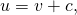contrary to Einstein’s postulates.

According to experimental results and the second postulate of relativity, light from the car’s headlights moves away from the car at speed c and toward the observer on the sidewalk at speed c.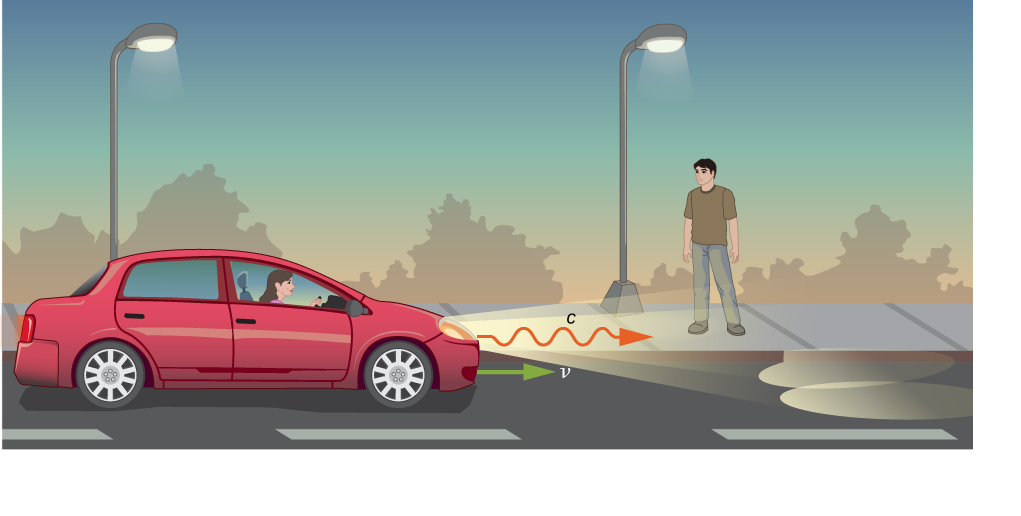Both the distance traveled and the time of travel are different in the two frames of reference, and they must differ in a way that makes the speed of light the same in all inertial frames. The correct rules for transforming velocities from one frame to another can be obtained from the Lorentz transformation equations.

### Relativistic Transformation of Velocity

Suppose an object P is moving at constant velocity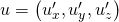as measured in the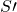frame. Theframe is moving along its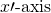at velocity v. In an increment of time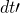, the particle is displaced by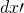along the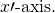Applying the Lorentz transformation equations gives the corresponding increments of time and displacement in the unprimed axes: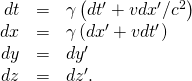The velocity components of the particle seen in the unprimed coordinate system are then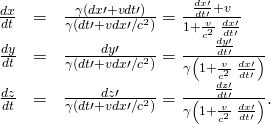We thus obtain the equations for the velocity components of the object as seen in frame S: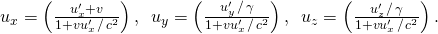Compare this with how the Galilean transformation of classical mechanics says the velocities transform, by adding simply as vectors: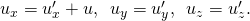When the relative velocity of the frames is much smaller than the speed of light, that is, when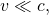the special relativity velocity addition law reduces to the Galilean velocity law. When the speed v ofrelative to S is comparable to the speed of light, the relativistic velocity addition law gives a much smaller result than the classical (Galilean) velocity addition does.

Velocity Transformation Equations for Light Suppose a spaceship heading directly toward Earth at half the speed of light sends a signal to us on a laser-produced beam of light ((Figure)). Given that the light leaves the ship at speed c as observed from the ship, calculate the speed at which it approaches Earth.

How fast does a light signal approach Earth if sent from a spaceship traveling at 0.500c?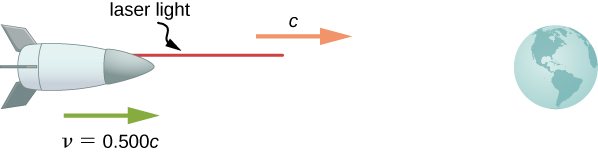Strategy Because the light and the spaceship are moving at relativistic speeds, we cannot use simple velocity addition. Instead, we determine the speed at which the light approaches Earth using relativistic velocity addition.

Solution

1. Identify the knowns: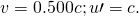2. Identify the unknown: u.
3. Express the answer as an equation: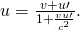4. Do the calculation: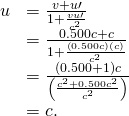SignificanceRelativistic velocity addition gives the correct result. Light leaves the ship at speed c and approaches Earth at speed c. The speed of light is independent of the relative motion of source and observer, whether the observer is on the ship or earthbound.

Velocities cannot add to greater than the speed of light, provided that v is less than c and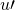does not exceed c. The following example illustrates that relativistic velocity addition is not as symmetric as classical velocity addition.

Relativistic Package Delivery Suppose the spaceship in the previous example approaches Earth at half the speed of light and shoots a canister at a speed of 0.750c ((Figure)). (a) At what velocity does an earthbound observer see the canister if it is shot directly toward Earth? (b) If it is shot directly away from Earth?

A canister is fired at 0.7500c toward Earth or away from Earth.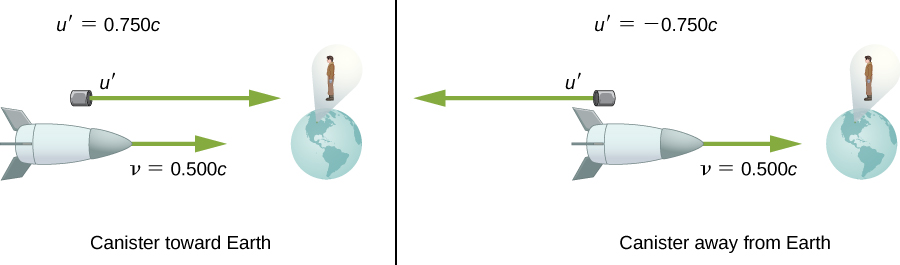Strategy Because the canister and the spaceship are moving at relativistic speeds, we must determine the speed of the canister by an earthbound observer using relativistic velocity addition instead of simple velocity addition.

Solution for (a)

1. Identify the knowns: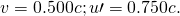2. Identify the unknown: u.
3. Express the answer as an equation:4. Do the calculation: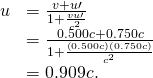Solution for (b)

1. Identify the knowns: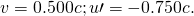2. Identify the unknown: u.
3. Express the answer as an equation:4. Do the calculation: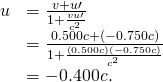Significance The minus sign indicates a velocity away from Earth (in the opposite direction from v), which means the canister is heading toward Earth in part (a) and away in part (b), as expected. But relativistic velocities do not add as simply as they do classically. In part (a), the canister does approach Earth faster, but at less than the vector sum of the velocities, which would give 1.250c. In part (b), the canister moves away from Earth at a velocity of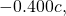which is faster than the −0.250c expected classically. The differences in velocities are not even symmetric: In part (a), an observer on Earth sees the canister and the ship moving apart at a speed of 0.409c, and at a speed of 0.900c in part (b).

Check Your Understanding Distances along a direction perpendicular to the relative motion of the two frames are the same in both frames. Why then are velocities perpendicular to the x-direction different in the two frames?

Although displacements perpendicular to the relative motion are the same in both frames of reference, the time interval between events differ, and differences in dt andlead to different velocities seen from the two frames.

### Summary

• With classical velocity addition, velocities add like regular numbers in one-dimensional motion: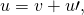where v is the velocity between two observers, u is the velocity of an object relative to one observer, andis the velocity relative to the other observer.
• Velocities cannot add to be greater than the speed of light.
• Relativistic velocity addition describes the velocities of an object moving at a relativistic velocity.

### Problems

If two spaceships are heading directly toward each other at 0.800c, at what speed must a canister be shot from the first ship to approach the other at 0.999c as seen by the second ship?

Two planets are on a collision course, heading directly toward each other at 0.250c. A spaceship sent from one planet approaches the second at 0.750c as seen by the second planet. What is the velocity of the ship relative to the first planet?

0.615c

When a missile is shot from one spaceship toward another, it leaves the first at 0.950c and approaches the other at 0.750c. What is the relative velocity of the two ships?

What is the relative velocity of two spaceships if one fires a missile at the other at 0.750c and the other observes it to approach at 0.950c?

0.696c

Prove that for any relative velocity v between two observers, a beam of light sent from one to the other will approach at speed c (provided that v is less than c, of course).

Show that for any relative velocity v between two observers, a beam of light projected by one directly away from the other will move away at the speed of light (provided that v is less than c, of course).

(Proof)

### Glossary

method of adding velocities when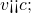velocities add like regular numbers in one-dimensional motion:where v is the velocity between two observers, u is the velocity of an object relative to one observer, andis the velocity relative to the other observer